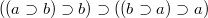﻿

### On a new proof completeness of p. i. Propositional Calculus

#### Abstract

In this paper, in order to obtain some interesting properties of the implicational algebras, we prove a completeness theorem on positive implicational Propositional Calculus in which we use the axiom schema$((a \supset b) \supset b) \supset ((b \supset a) \supset a)$ in an essential manner. We demonstrate this theorem without using neither Zorn’s Lemma, nor similar properties.

DOI Code: 10.1285/i15900932v27n1p139

Keywords: Implication; Algebras

Full Text: PDF PS